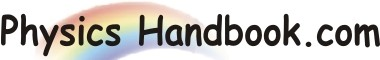HOME TOPICS DEFINITIONS TABLES LAWS INVENTIONS EXPERIMENTS QUIZ VIDEOS
 Newton's Second Law The acceleration produced by a particular force acting on a body is directly proportional to the magnitude of the force and inversely proportional to the mass of the body. i.e.    F  =  ma where, F  =  Force           m  =  Object's Mass           a   =  Object's Acceleration Newton's Second Law of Motion defines the relationship between acceleration, force, and mass. For example, If you apply a force to an empty shoebox sitting on a table, the shoebox applies an equal force back on you. Because your mass is much larger than the mass of the shoebox, the force you exert causes it to accelerate away from you and the force it exerts on you wouldn't cause much acceleration at all.